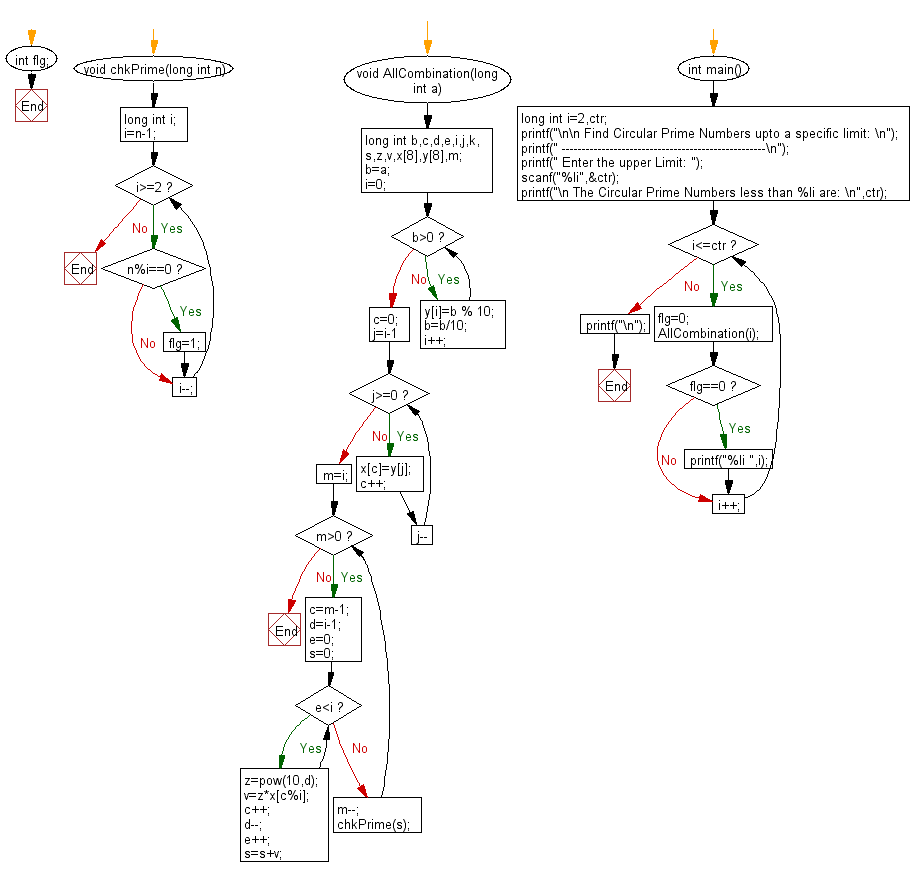﻿ C Program: Find circular prime numbers upto a specific limit - w3resource# C Exercises: Find circular prime numbers upto a specific limit

## C Numbers: Exercise-28 with Solution

Write a program in C to find circular prime numbers upto a specific limit.

Test Data
Enter the upper Limit: 1000

Sample Solution:

C Code:

``````# include <stdio.h>
# include <stdlib.h>
# include <stdbool.h>
# include <math.h>

int flg;
void chkPrime(long int n)
{
long int i;
i=n-1;
while(i>=2)
{
if(n%i==0)
{
flg=1;
}
i--;
}
}

void AllCombination(long int a)
{
long int b,c,d,e,i,j,k,s,z,v,x,y,m;
b=a;
i=0;
while(b>0)
{
y[i]=b % 10;
b=b/10;
i++;
}
c=0;
for(j=i-1;j>=0;j--)
{
x[c]=y[j];
c++;
}
m=i;
while(m>0)
{
c=m-1;
d=i-1;
e=0;
s=0;
while(e<i)
{
z=pow(10,d);
v=z*x[c%i];
c++;
d--;
e++;
s=s+v;
}
m--;
chkPrime(s);
}
}

int main()
{
long int i=2,ctr;

printf("\n\n Find Circular Prime Numbers upto a specific limit: \n");
printf(" ---------------------------------------------------\n");
printf(" Enter the upper Limit: ");
scanf("%li",&ctr);
printf("\n The Circular Prime Numbers less than %li are: \n",ctr);
while(i<=ctr)
{
flg=0;
AllCombination(i);
if(flg==0)
{
printf("%li ",i);
}
i++;
}
printf("\n");

}
```
```

Sample Output:

``` Enter the upper Limit: 1000

The Circular Prime Numbers less than 1000 are:
2 3 5 7 11 13 17 31 37 71 73 79 97 113 131 197 199 311 337 373 719 733 919 971 991
```

Pictorial Presentation:Flowchart:C Code Editor:

What is the difficulty level of this exercise?

Test your Programming skills with w3resource's quiz.

﻿

## C Programming: Tips of the Day

Why do C and C++ compilers allow array lengths in function signatures when they're never enforced?

It is a quirk of the syntax for passing arrays to functions.

Actually it is not possible to pass an array in C. If you write syntax that looks like it should pass the array, what actually happens is that a pointer to the first element of the array is passed instead.

Since the pointer does not include any length information, the contents of your [] in the function formal parameter list are actually ignored.

Ref : https://bit.ly/3fhlvdH